Math Concepts
What are integers and why it is important?
15.1k views

 1 Introduction 2 Definition of Integers 3 Operations on Integers 4 Properties of Integers 5 Summary 6 About Cuemath 7 Frequently Asked Questions (FAQs) 8 External References

Jan 8 2021

## Introduction

There are many different kinds of numbers that we come across in algebra. Some of these kinds of numbers are related to each other.

Before we learn how these numbers are related to each other, we need to learn about them separately. In this article, you will learn about integers and their operations.

Whenever you go to another country, you will have to exchange your currency for those countries’ currency. Not only that, the value of your currency may either increase or decrease in different countries.

Because each country has different values in relation to their currency.

These positive and negative values are the building blocks of mathematics. On the number line they are represented as follows:-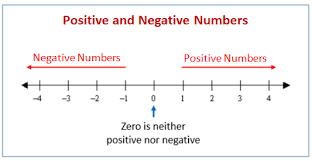These numbers have many uses in real life as well as in math class. You will better understand with the following examples:

1. Money that we receive from somewhere as salary or gifts is added to our account and the money that we give someone is subtracted from our account.1. When you get your blood pressure checked. It may come out to be high or low. A value that is above normal pressure is called positive and a value that is below normal is called negative.## Definition of Integers

Integers are the natural numbers, the negatives of these numbers, or zero. An integer is a complete entity. Integers are the numbers that can be positive, negative, or zero, numbers with no fractional part (no decimals).

The examples of integers are, 1, -2, 7, -8, 9, -12, etc.

The collection of all integers is represented by the symbol “Z”.

Some more examples:
If someone is overweight than normal then it will be represented as positive and if someone is underweight than normal then it is represented as negative.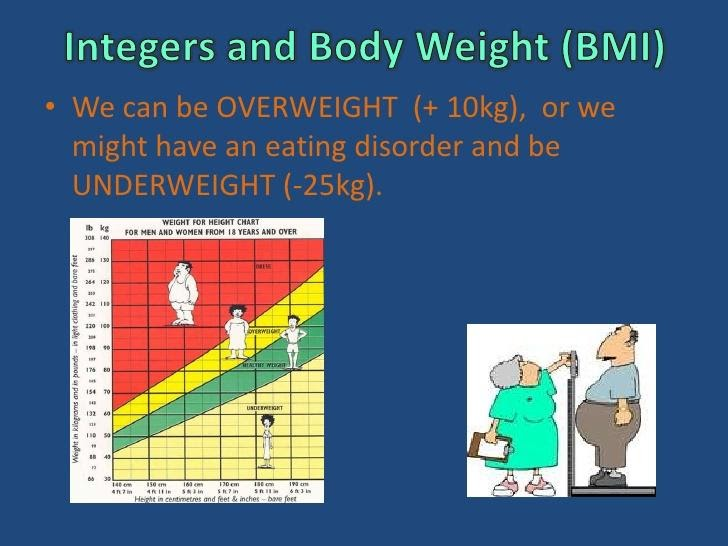For example, $$–2$$ is an integer.
$$–2$$ is read as ‘negative two’.
This can also be written as $$–2$$ or $$(​​-2)$$
It is 2 less than 0.
$$0 – 2 = –2$$.
So natural numbers (which lie on the right side of zero) are positive, their counterparts (negative natural numbers) are negative.

We can use the number line to compare two integers.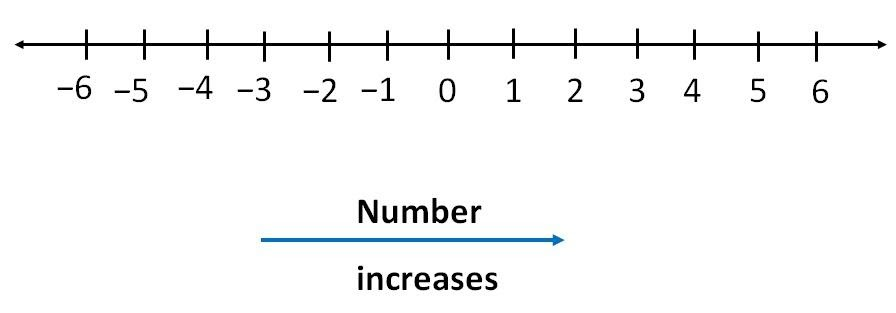We say that –3 is ‘greater than’ –6 because –3 lies to the right of –6 along the line.
Similarly, –5 ‘is less than’ 2 because –5 lies to the left of 2.

So we conclude that if a number lies on the left side it will be smaller than the number which lies on the right side.

Example: Which of the following options is not true with respect to a given number line?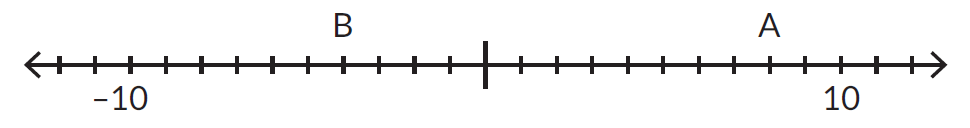(a) B is greater than –10
(b) A is greater than 0
(c) A is greater than B
(d) B is greater than 0

Answer: The first three options are correct but B lies on the left side of 0, so it is not greater than 0. So clearly option (D) is not true.

 (d) B is greater than 0

Example: The six-day forecast for Antarctica lists the temperatures (in Celsius) as –52°C, –53°C, –40°C, –58°C, –70°C, –79°C. Which choice shows the temperatures in order from the lowest to the highest?
(A) –79°C, –70°C, –52°C, –53°C, –40°C, –58°C
(B) –52°C, –53°C, –58°C, –70°C, –79°C, –40°C
(C) –79°C, –70°C, –58°C, –53°C, –52°C, –40°C
(D) –40°C, –52°C, –53°C, –58°C, –70°C, –79°C

Answer: On the number line, we see that the larger is the negative value the smaller is the number. Using this, we can say option (C) is correct.

 (C) –79°C, –70°C, –58°C, –53°C, –52°C, –40°C

## Operations on Integers

Integers are used to perform various arithmetic operations, like addition, subtraction, multiplication, and division.  Let us understand them one by one.

### Addition and Subtraction of Integers

$$(+) + (+) = +$$
$$(-) + (-) = –$$

Subtraction rule:
$$(+) – (+) = (+) + (-)$$; consider the sign of a greater number
$$(-) – (-) = (-) + (+)$$; consider the sign of a greater number
$$(+) – (‐) = (+) + (+)$$; the answer will be positive
$$(‐) – (+) = (-) + (-)$$; the answer will be negative

Note: We can always put the sign of the greater number in the answer.

Let’s understand with movement on the number line. I am representing a positive movement with blue (right side) and a negative movement with red (left side).

Consider the following examples where we start from the first number. If a positive number is added we move towards the right and if a negative number is added we move towards the left: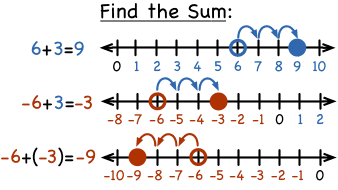The last example of negative added to negative can also be understood with the following example in which we move towards the left for both the negative numbers.

So $$(-2) + (-5) = (-7)$$ can be understood as follows:-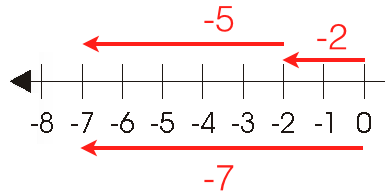Example: Which of the following number lines represents $$–1 –4$$?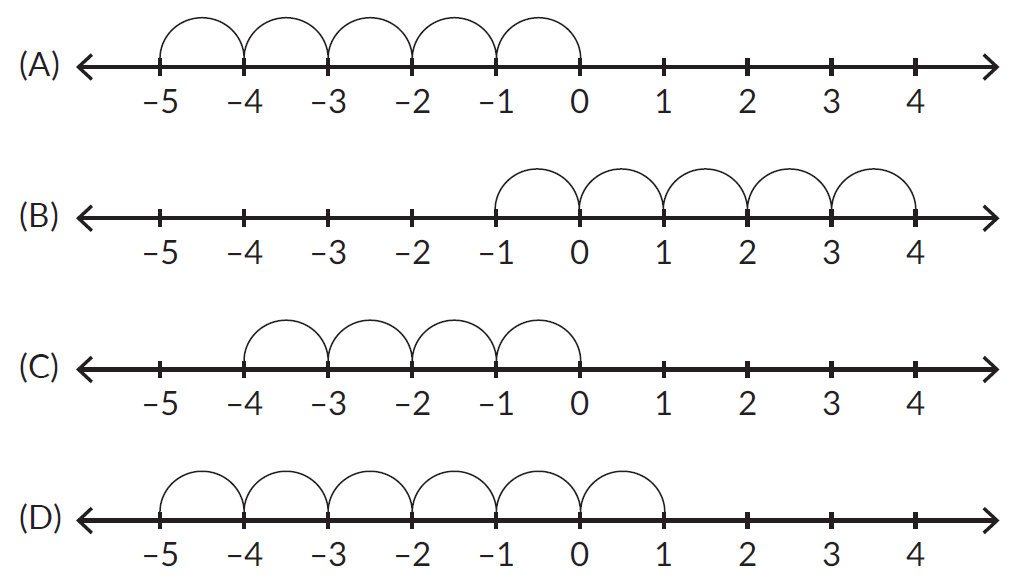Answer: From the above illustrations, it is clear that option (A) is correct.

Example: The difference between the sum of all the even numbers and the sum of all the odd numbers between 10 and 20 is ______.
(A) –12         (B) –14         (C) –15         (D) –16

Answer: Sum of all even numbers: $$12 +14 +16 + 18 = 60$$
Sum of all odd numbers: $$11 + 13 + 15 + 17 + 19 = 75$$
So the required difference is $$60 – 75 = – 15$$.

So option (C) is correct.

 (C) –15

Example: The distance above the ground is represented by positive integers and the distance below the ground by negative integers. An elevator begins to descend from 15 m above the ground at a rate of 5 m per minute. What will be its position after 45 minutes?
(A) 210 m         (B) –210 m     (C) 215 m         (D) –225 m

Answer: The distance traveled is calculated as
Distance = speed x time = $$5 * 45 = 225 m$$
But since the elevator is descending so the distance travelled will be taken negative and we calculate the position of the elevator as
$$15 + (-225) = -215 m$$.
Thus after 45 minutes, the elevator will be at 215 m below the ground level.

 (C) 215 m

### Multiplication and Division of Integers

Integers follow the following rules for multiplication and division.

Multiplication and Division rules:
$$(+) * (+) = +$$ and $$(+) ÷ (+) = +$$
$$(-) * (-) = +$$ and $$(-) ÷ (-) = +$$
$$(+) * (-) = –$$ and $$(+) ÷ (-) = -$$
$$(-) * (+) = –$$ and $$(-) ÷ (+) = -$$

Notice that the rule for the division of integers is the same for multiplying integers. Therefore, if you remember the rule for multiplying the integers, you already know it for the division of integers also.

This can also be learned as the same signs will have a positive answer and opposite signs will have a negative answer.

And for more than two numbers in multiplication or/and division, we can say if negative comes an odd number of times then the answer will be negative and if a negative sign comes an even number of times then the answer is positive.

As with whole numbers, consider multiplication as repeated addition. Using the number line, we make multiple jumps of a given size. For example, the following picture shows the product $$3 * 4$$ as 3 jumps of 4 units each.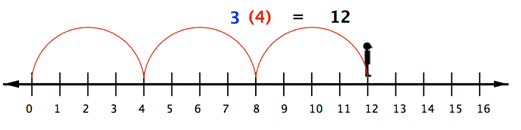Similarly, to multiply integers like $$3 * (−4)$$, we can face left (toward the negative side) and make three “jumps” forward (in a negative direction).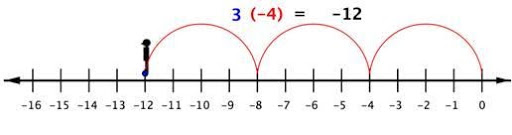The division can also be understood as $$-12÷3=-4$$.

Example: In a class test of 20 questions, 5 marks are given for every correct answer, and $$(–2)$$ marks are given for every incorrect answer. Kavya attempts all questions and gets only 7 of them correct. What is Kavya's score?

Answer: No. of correct questions = 7, this gives a score of $$7 * 5 = 35$$.
No. of incorrect questions = 13, this gives a score of $$13 * (–2) = (–26)$$
Thus Kavya’s total score =$$35 + (–26) = 9$$.

 The total score of Kavya = 9

## Summary

Integers are numbers that are positive, negative, and zero but not fractions/decimals. All mathematical operations can be applied to integers. Signs of the two numbers on which we apply any operation can affect the answer.

I hope the article has helped you in understanding integers and their operations.

Integers in real life?

• Real-life integers are used to check accounts
• If there are sufficient funds, it represents positive numbers.
• If money is overdrawn from the account, it represents negative numbers.
• If there is a profit, we have positive numbers.
• If there is a loss, we have negative numbers.

Cuemath, a student-friendly mathematics platform, conducts regular Online Classes for academics and skill-development, and their Mental Math App, on both iOS and Android, is a one-stop solution for kids to develop multiple skills. Understand the Cuemath Fee structure and sign up for a free trial.